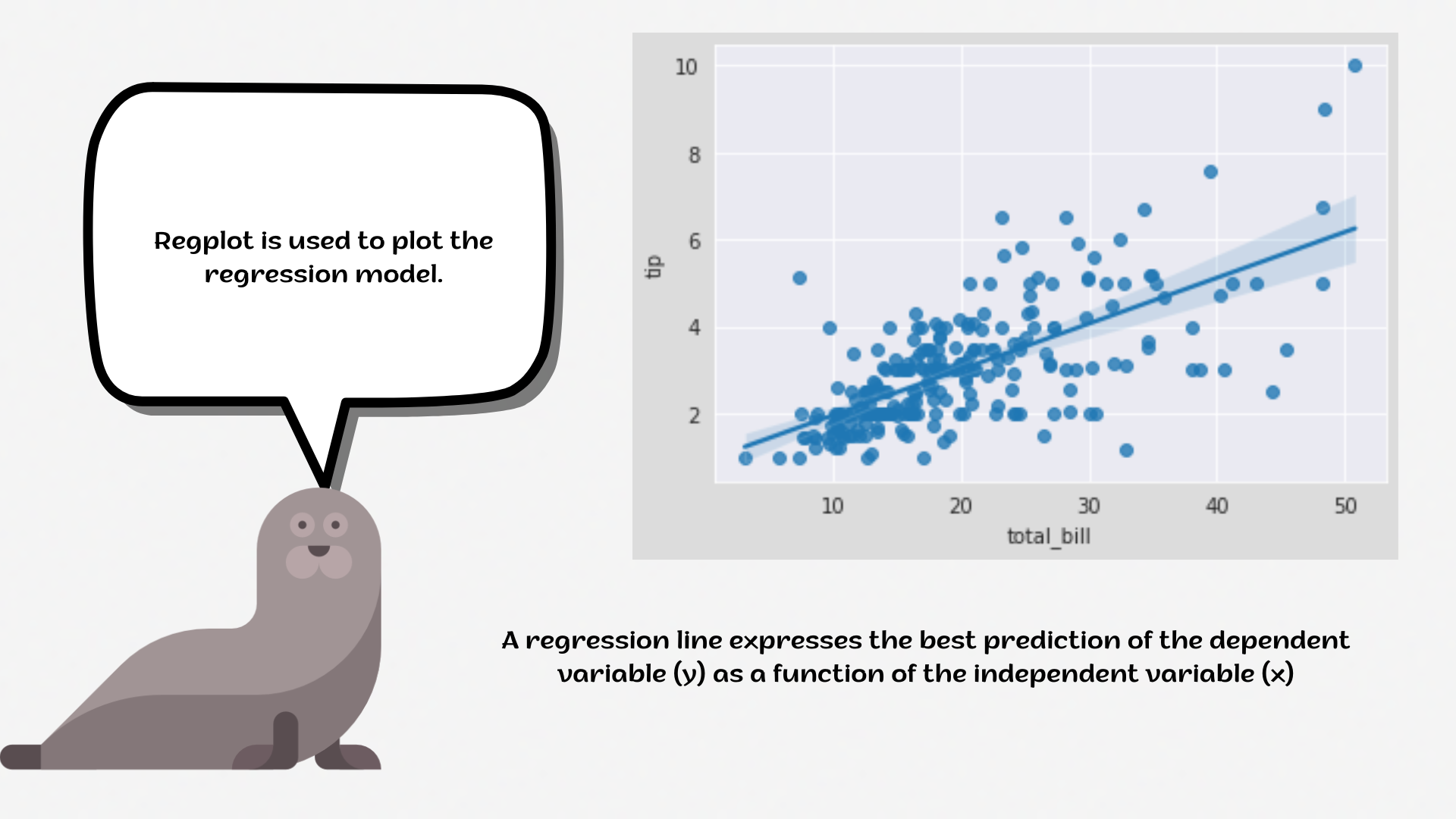Course Content

# Deep Dive into the seaborn Visualization

1. Light Start

2. Distributions of Data

3. Categorical Plot Types

4. Matrix Plots

5. Multi-Plot Grids

6. Regression Models

Deep Dive into the seaborn Visualization

##RegplotThe `regplot` is used to plot data and a linear regression model fit. There are a number of mutually exclusive options for estimating the regression model.1. Set the `'darkgrid'` style with the `'tan'` figure facecolor and `'cornsilk'` `axes .facecolor`.
2. Create a `regplot` using the `seaborn` library:
• Set the `x` parameter equals the `'total_bill'`;
• Set the `y` parameter equals the `'tip'`;
• Set the `marker` parameter equals the `'+'`;
• Set the `'green'` `color`;
• Disable the `fit_reg` parameter;
• Set the data;
• Display the plot.

Everything was clear?

Section 6. Chapter 1